Interstitial Flow in Cancerous Tissue: Effect of Considering Remodeled Capillary Network | OMICS International
Journal of Tissue Science & Engineering

Like us on:

# Interstitial Flow in Cancerous Tissue: Effect of Considering Remodeled Capillary Network

Visit for more related articles at Journal of Tissue Science & Engineering

#### Abstract

Interstitial fluid flow has been studied by many cancer researchers for investigation of how interstitial flow can
affect drug delivery. This paper couples blood flow through a capillary network induced by tumor angiogenesis and
fluid flow in interstitium and investigates the effects of consideration of a remodeled network and adaptability of
capillary on interstitial flow. Two approaches are studied for showing the remodeling effects. In the first approach,
the capillary network is presumed to have capillary with constant diameter. For the second approach, the adaptable
capillaries are assumed in which the metabolic and hemodynamic stimuli changes capillary’s diameter based on
sending signals to capillary. Numerical results show that the intravascular pressure has more uniform distribution
along the network and capillary diameter has greater value in the network. The prediction of interstitial pressure for
the second approach shows higher values compared to the first approach. Results from interstitial flow and blood
flow distribution in normal and cancerous tissues have beneficial information about prediction of successive drug
delivery.

 Keywords Tumor induced angiogenesis; Capillary network; Remodeling; Blood flow in capillary network; Interstitial fluid flow Introduction The abnormal and complicate vasculature induced by solid tumor is introduced as a main reason of unsuccessful drug delivery to tumors. The high interstitial pressure because of high permeability of vessel in tumor region is another barrier to drug transport. The mathematical model used for solid tumor simulation predicts mechanism of drug delivery to tumor cells and helps to find better methods for drug delivery [1,2]. Solid tumor growth modeling involves many complicated processes on a wide range of spatial and temporal scales. The multi-temporal nature of tumor involves formation of vessels induced by angiogenesis which changes in a scale of days and fluid flow and capillary adaptation which occurs in a scale of seconds. The mathematical model of tumor consists of blood flow convection in micro vessel at the micro scale to interstitial fluid flow which includes the convection and diffusion mechanism at the largest scale . The fluid flow modeling in solid tumor as a porous medium by neglecting small scales is common. Soltani et al.  introduced the critical tumor radius and critical necrotic radius based on results of fluid flow in tumor done by developed mathematical model of interstitial fluid flow. The effect of shape and size of tumor in interstitial fluid flow is investigated by Soltani et al. . In these works, the capillary network is substituted by uniform distribution of mass source. The fluid flow simulation in tumor induced vascular network is investigated in many studies. In some research the blood flow is considered only in a single capillary while in other studies, blood flow through a capillary network is coupled with fluid flow in tumor tissue [6-10]. In the latter case, capillary network is generated by mathematical model or image processing from experimental data [6,7,9,10]. None of the above works however considered the non-continuous behavior of blood through capillaries or adaptability of capillary’s diameter. Not only chemical responses from tumor growth but also functional needs affect the capillary network structure induced by angiogenesis. The functional needs applied in this study are hemodynamic stimuli implemented by blood flow and metabolic stimuli exerted by tissue, respectively. To simulate the structural adaptation of vascular network, a mathematical model based on experimental observation is used. This mathematical model is developed by Pries et al. [11,12]. They investigated structural adaptation of capillary network by a theoretical method. They set metabolic condition and the wall shear stress induced by blood flow and or intravascular pressure as minimal requirements to have realistic blood flow in capillary network. In some studies, the blood flow through capillary network in tumor modeled by considering adaptability of capillary and blood behavior in capillaries [13-17]. In spite of the valuable body of work, the comprehensive model which includes remodeling tumor induced capillary network with interaction by interstitial flow is not considered. In the previous work in our group a mathematical model was developed that couples interstitial fluid flow and intravascular fluid flow at the same time by considering adaptability of capillary’s radius to signals from hemodynamic and metabolic stimuli . In the present work, the simulation method presented in our previous work is developed by adding lymphatic system in the mathematical model of interstitial fluid flow, and statistical analysis . Results of mathematical and statistical analysis of two approaches, capillary network with rigid vessels and capillary network with remodeled structure, are compared. The detail of both approaches is introduced in Soltani and Chen . Results show that fluid flow modeling through network with adaptable capillaries has realistic distribution of vessel radii, uniform blood flow, and elevated intravascular pressure in the whole network. This prediction is lead to higher interstitial pressure levels in remodeled network compared to interstitial pressure in the network with rigid capillaries. Materials and Methods Angiogenesis, mathematical model The present Angiogenesis model is motivated by the tumor-induced angiogenesis model initially proposed by Anderson and Chaplain . This mathematical model predicts the capillary formation by tracking the endothelial cells motion in capillary sprout tips. The detail of rules for sprouting angiogenesis and algorithm of this method is determined in our previous work Anderson and Chaplain and Soltani and Chen [18,19]. Remodeling capillary network The adaptability of capillary’s radius is simulated by mathematical model introduced by Pries et al. [11,20]. The changes of radius are calculated by Pries et al. :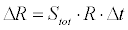(1) Stot includes the influences of the hemodynamic (Sh), and a metabolic mechanism (Sm):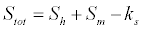(2) Where Sh: introduced as hemodynamic stimuli and defined by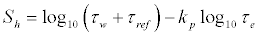(3) Sm: Metabolic stimuli and defined by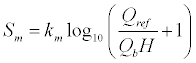(4) Where τw : the wall shear stress in capillary induced by flow rate,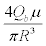τref : A constant value for preventing singular behavior logarithm function τe : The wall shear stress induced by the intravascular pressure,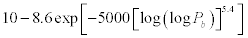Qref : The largest value of Qb in the network ks : Shrinking stimuli kp, km: Positive real numbers The detail of these stimulus and their effects are mentioned in our previous work . Intravascular pressure calculation The blood flow through micro vessel network is modeled similar to electrical network. Since blood flow rate in micro vessel network is equal to electric current in electrical network, the balance of blood flow in each interconnecting point like c can be implemented same as balance of electric current in electrical networks (Figure 1):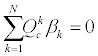(5) Where N: The number of adjacent nodes βk : ‘1’ shows connection with adjacent node and ‘0’ shows no connection with adjacent node.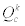: The blood flow rate through each capillary (Figure 2), and calculated as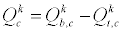(6) Where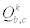: Blood flow through each capillary,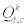: The flow rate across the microvessel wall or transvascular flow. For blood flow in capillary tubes, Poiseuille’s law can be applied: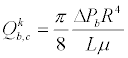(7) Where R: the vessel radius, L: the vessel length, μ: blood viscosity, ΔPb: calculated by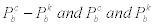are corresponding blood pressure at each node. The transvascular flow rate is calculated by Starling’s law [21,22]: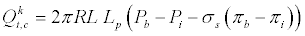(8) where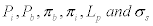are the interstitial fluid pressure, blood pressure in capillary, capillary oncotic pressure, interstitial oncotic pressure, hydraulic conductivity, and osmotic reflection coefficient, respectively. The fluid flow governing equation for a porous medium is used to calculate the interstitial pressure for peripheral tissue of a vascular network. The continuity equation for incompressible fluid flow in the porous media with source and sink of mass is :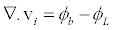(9) Where vi: the interstitial fluid velocity, φb : theflow rate from vessel to interstitium or vice versa, φL : theflow rate from interstitium to lymphatic system. The starling’s law represents the fluid flow rate across the microvessel wall .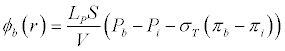(10) The lymphatic drainage term is: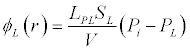(11) Where PL: The lymphatic pressure. φL is considered only in normal tissue and in tumor tissue its value is zero . The general form of momentum equation for porous media is as follows :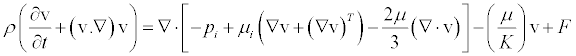(12) Where K: the permeability of the porous medium, ρ: the density of interstitium media, μi: the viscosity of interstitium media, F: influence of gravity and other volume forces. By considering interstitial flow as Newtonian fluid and low velocity for fluid flow and neglecting the friction, equation (12) is simplified to Darcy’s law: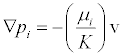(13) The K/μi is defined as interstitium hydraulic conductivity κ. By coupling Equations (9) and (13), considering source term wherever there is a capillary and uniform distribution of sink term, the governing equation for interstitial flow is obtained as follows (For more detail see ):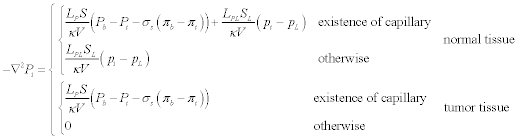(14) Blood behavior in microvessel The dependency of blood viscosity in microvessel to radius and hematocrit introduced as Fahraeus–Lindqvist effect and independency of hematocrit fraction into daughter vessels to blood flow fraction are known as non-continuous behavior of blood . The dependency of viscosity to radius is shown in Figure 3. The detail of non-continuous behavior modeling is mentioned in our previous work . Calculation algorithm Intravascular (Pb) and Interstitial (Pi) pressures are coupled to each other based on Equation (8). Therefore, Equations (5) and (14) should be solved at the same time to find the blood pressure in capillary network and interstitial fluid pressure. The numerical method is used for solution these equations. The advanced mathematical method is applied to discretize the interstitial fluid flow equation (equation 14) . The general algorithm used in this work is shown in Figure 4. In the first approach, rigid network, the hematocrit, viscosity, and vessel radius are assumed to be constant during simulation. For the second approach, the mentioned parameters are assumed to update during simulation. The detail of numerical procedure is described in Soltani and Chen . Boundary and initial condition and model parameterization The 2D computational domain (Figure 5), in which parent vessel is located in the left and tumor in the right, is considered for simulation. The radius of circular tumor is assumed 0.4 of domain length. Boundary and initial conditions Intravascular flow: To carry out the intravascular flow simulation, pressure values for the inlet and outlet of parent vessel are required. Taking into account the physiological condition at the microvessel scale, the 3325Pa (25 mmHg) for inlet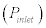and 1330Pa (10 mmHg) for outlet pressures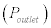is considered. The initial value of Pb is considered to be 1330 Pa. The initial radius of each capillary segment for simulation with remodeling method is assumed to be 6 μm. For the parent vessel the radius is 14 μm which stays constant during the remodeling procedure . For simulation with rigid capillary, the vessel radius is prescribed with a method presented by Wu et al.  as follows: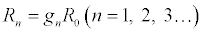(15) Where n is the value of branching generation, R0 is the radius of the parent vessels, Rn is the radius of the nth generation of vessels, gn is the ratio coefficient smaller than 1. gn in equation (15), given by Wu et al.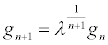(16) In which is 0.65 and g0 is 0.8. The radius of vessels is remained constant during simulation. Interstitial flow: The boundary condition used in solving of Equation (14) is shown in Figure 5. Anon-flux (symmetry) boundary is applied in right, down and upper edges, i.e.,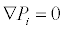(17) For the left boundary, the interstitial pressure is constant; the Dirichlet type of boundary condition is applied : Pi = 0 for outer region (18) The continuity of pressure and velocity for Equation (14) are considered as appropriate boundary conditions for boundary between normal and tumor tissue: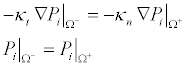(19) Where Ω− and Ω+ indicate the tumor and normal tissue at the outer edge of the solid tumor; the t and n are the normal and tumor tissue, respectively. Model parameters: The material properties for normal and tumor tissue listed in Table 1 and used in interstitial fluid flow calculation are taken from our previous work . The parameters used for blood flow simulation through microvessel network are listed in Table 2. Results and Discussion Two methods are used for simulation of blood flow in capillary network and peripheral tissues. Results of the first method are obtained by simulating fluid flow in a network induced by tumor angiogenesis with constant values for blood rheology and vessel radius. In the second method, results are obtained using the proposed realistic model assuming the Fahraeus–Lindqvist effect of blood viscosity and remodeling of the capillary network. The capillary network used in this paper is generated by discrete angiogenesis method with considering 6 endothelial cells in parent vessel. The capillary network shown in Figure 6 (blue line) is used in numerical simulation of intravascular and interstitial pressure. The pruned networks are illustrated in Figure 6 with green lines. Resulted networks agree well qualitatively with the experiments [27,28]. For a better comparison of the results of two approaches, the interstitial pressure is non-dimensionalized. The non-dimensional interstitial pressure is defined by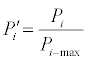(20) In which Pi-max is the maximum interstitial pressure calculated for two approaches. The calculation shows that the maximum pressure belongs to the remodeled network which is around 1100 Pa. Also, the x and y positions are non-dimensionalized by length of the domain. The 3D graphs of interstitial pressure for two approaches are shown in Figures 7 and 8. At the tumor center, the highest value of interstitial pressure is occurred. The interstitial pressure decreased rapidly towards the periphery. The higher vessel permeability and lack of lymphatic system in the tumor region caused the high elevation of interstitial pressure near the tumor. This effect is observed in our previous works Soltani and Chen and Jain et al. [4,5,29], and in the experimental results of Arifin et al. , Huber et al.  and Heine et al. . The interstitial velocity contour is shown in Figures 9 and 10 for two calculation methods. The maximum value of IFV is close to the boundary between normal and tumor tissue. Since the IFV based on Equation (13) is only depended to IFP gradient and the IFP has uniform value in tumor region, the IFV is zero. The maximum value of IFV is in the same order as the prediction of Jain et al.  and experimental observation of Hompland et al. . The second approach-remodeled network-predicts the highest interstitial pressure approximately 150% of the highest value of interstitial pressure in rigid network. Results presented in the following figures show how considering realistic condition such as remodeling and blood behavior in capillary can affect on interstitial pressure. The blood flow distribution for both rigid and remodeled networks is shown in Figures 11 and 12. The comparison of blood flow for two networks shows that blood flow in remodeled network has a uniform distribution than the blood flow in rigid capillaries. The pruning method based on low flow rate is used in blood flow simulation. This method is based on the experimental observation in which the vessels collapse in low flow and shear stress . Because the capillaries far from parent vessel in rigid network receive low blood flow rate, most of them are eliminated in pruning process. However, in remodeled network, most of these pruned capillaries are retained. The considering structural adaptation in modeling process decreases flow resistance through the capillaries, and also prevents shunt fluid path ; therefore, vessels far from parent vessel get more blood flow rate. Figures 13 and 14 show the radius distribution in the domain. The statistical analysis of radius distribution is shown in Figures 15 and 16. The density of each radius is calculated and shown for two approaches. As shown in these figures, the number of great radius sizes in remodeled network is much more than that of rigid network. The bigger radius in remodeled network decreases resistance flow in the network and makes uniform distribution blood flow as illustrated in Figure 12. The uniform distribution of blood flow pattern and radius size affect on intravascular pressure. Figures 17 and 18 show the intravascular distribution for two cases. The average intravascular pressure at each constant x in domain for two approaches is shown in Figure 19. Figure 19 shows that the intravascular pressure from the parent vessel to tumor has a more uniform value for remodeled network in comparison to that of rigid network. Also, an intravascular pressure distribution obtained statistically (Figure 20) shows that the remodeled network has a larger density in higher pressure values in comparison to rigid network. These results show why the remodeled network predicts higher interstitial pressure than rigid network. The elevated intravascular pressure and high leaky microvessels in tumor region increase the transvascular flow rate and subsequently the interstitial pressure as shown in Figures 7 and 21. Conclusion This study provides a numerical simulation of interstitial fluid flow in normal and tumor tissues with distribution of intravascular pressure based on tumor-induced vascular network in two situations: a rigid vascular network and remodeled capillary network. The proposed method couples different simple models to describe processes ranging from flow through porous media, angiogenesis, blood viscosity in capillaries, phase separation, and structural adaptation of microvessel network. Then it is discussed how these simple methods incorporate important simplifications to complex processes and lead to more realistic results. The adaptability of capillaries in response to hemodynamic and metabolic stimuli which remodels network structure leads to greater capillary radius compared to the rigid capillary network model. The more-uniform blood flow distribution caused by greater capillary radius results in low resistance fluid flow and higher intravascular pressure. This elevated intravascular pressure in immature blood vessels for remodeled network leads to higher interstitial pressure prediction than interstitial pressure prediction for rigid network. References Jain RK (2001) Normalizing tumor vasculature with anti-angiogenic therapy: a new paradigm for combination therapy. Nat Med 7: 987-989. Pozrikidis C (2010) Numerical simulation of blood and interstitial flow through a solid tumor. J Math Biol 60: 75-94. Soltani M, Chen P (2013) Numerical Modeling of Interstitial Fluid Flow Coupled with Blood Flow through a Remodeled Solid Tumor Microvascular Network. PLoS One 8: e67025. Soltani M, Chen P (2011) Numerical modeling of fluid flow in solid tumors. PLoS One 6: e20344. Soltani M, Chen P (2012) Effect of tumor shape and size on drug delivery to solid tumors. J Biol Eng 6: 4. McDougall SR, Anderson AR, Chaplain MA, Sherratt JA (2002) Mathematical modelling of flow through vascular networks: implications for tumour-induced angiogenesis and chemotherapy strategies. Bull Math Biol 64: 673-702. Stephanou A, Mcdougall S, Anderson A, Chaplain M (2005) Mathematical modelling of flow in 2D and 3D vascular networks: Applications to anti-angiogenic and chemotherapeutic drug strategies. Math Comput Model 41:1137-1156 Zhao G, Wu J, Xu S, MW Collins, Quan Long, et al (2007) Numerical simulation of blood flow and interstitial fluid pressure in solid tumor microcirculation based on tumor-induced angiogenesis. Acta Mech Sin 23: 477-483. Wu J, Long Q, Xu S, Padhani AR, Jiang Y (2008) Simulation of 3D solid tumour angiogenesis including arteriole , capillary and venule. Mol Cell Biomech 5: 1-23. Chauhan VP, Stylianopoulos T, Martin JD, PopoviÄ‡ Z, Chen O, et al. (2012) Normalization of tumour blood vessels improves the delivery of nanomedicines in a size-dependent manner. Nat Nanotechnol 7: 383-388. Pries AR, Secomb TW, Gaehtgens P (1998) Structural adaptation and stability of microvascular networks: theory and simulations. Am J Physiol 275: H349-360. Pries AR, Reglin B, Secomb TW (2001) Structural adaptation of microvascular networks: functional roles of adaptive responses. Am J Physiol Heart Circ Physiol 281: H1015-1025. McDougall SR, Anderson AR, Chaplain MA (2006) Mathematical modelling of dynamic adaptive tumour-induced angiogenesis: clinical implications and therapeutic targeting strategies. J Theor Biol 241: 564-589. Alarcón T, Byrne HM, Maini PK (2003) A cellular automaton model for tumour growth in inhomogeneous environment. J Theor Biol 225: 257-274. Alarcón T, Owen MR, Byrne HM, Maini PK (2006) Multiscale Modelling of Tumour Growth and Therapy: The Influence of Vessel Normalisation on Chemotherapy. Comput Math Methods Med 7: 85-119. Owen MR, Alarcón T, Maini PK, Byrne HM (2009) Angiogenesis and vascular remodelling in normal and cancerous tissues. J Math Biol 58: 689-721. Stephanou A, Mcdougall S, Anderson A, Chaplain M (2006) Mathematical modelling of the influence of blood rheological properties upon adaptative tumour-induced angiogenesis. Math Comput Model 44: 96-123 Anderson AR, Chaplain MA (1998) Continuous and discrete mathematical models of tumor-induced angiogenesis. Bull Math Biol 60: 857-899. Soltani M, Chen P (2013) Effect of Matrix Density and Matrix Degrading Enzymes in Continuous and Discrete Mathematical Models of Angiogenesis. J Comput Sci Pries AR, Reglin B, Secomb TW (2001) Structural adaptation of vascular networks: role of the pressure response. Hypertension 38: 1476-1479. Fung YC (1986) Blood rheology in microvessels. In: He, X.X. (Ed.), Biomechanics-Mechanical Properties of Living Tissues. Hunan Science Technique Publisher, China 157-197 Salathe EP, An KN (1976) A mathematical analysis of fluid movement across capillary walls. Microvasc Res 11: 1-23. Baxter LT, Jain RK (1989) Transport of fluid and macromolecules in tumors. I. Role of interstitial pressure and convection. Microvasc Res 37: 77-104. Vafail K (2011) POROUS Media, Application in Biological systems and biotechnology. CRC Press, USA. Toksvang LN, Berg RM (2013) Using a classic paper by Robin Fahraeus and Torsten Lindqvist to teach basic hemorheology. Adv Physiol Educ 37: 129-133. Ciofalo M, Collins MW, Hennessy TR (1999) Microhydrodynamics phenomena in the circulation. Mala, N. (Ed.), Nanoscale Fluid Dyn Physiol Process A Rev Study, WIT Press, Southampton, 219–236. Gimbrone MA Jr, Cotran RS, Leapman SB, Folkman J (1974) Tumor growth and neovascularization: an experimental model using the rabbit cornea. J Natl Cancer Inst 52: 413-427. Muthukkaruppan V, Kubai L, Auerbach R (1982) Tumor-induced neovascularization in the mouse eye. J Natn Cancer Inst 69: 699-708. Jain RK, Tong RT, Munn LL (2007) Effect of vascular normalization by antiangiogenic therapy on interstitial hypertension, peritumor edema, and lymphatic metastasis: insights from a mathematical model. Cancer Res 67: 2729-2735. Arifin DY, Lee LY, Wang CH (2006) Mathematical modeling and simulation of drug release from microspheres: Implications to drug delivery systems. Adv Drug Deliv Rev 58: 1274-1325. Huber PE, Bischof M, Jenne J, Heiland S, Peschke P, et al. (2005) Trimodal cancer treatment: beneficial effects of combined antiangiogenesis, radiation, and chemotherapy. Cancer Res 65: 3643-3655. Heine M, Freund B, Nielsen P, Jung C, Reimer R, et al. (2012) High interstitial fluid pressure is associated with low tumour penetration of diagnostic monoclonal antibodies applied for molecular imaging purposes. PLoS One 7: e36258. Hompland T, Ellingsen C, Øvrebø KM, Rofstad EK (2012) Interstitial fluid pressure and associated lymph node metastasis revealed in tumors by dynamic contrast-enhanced MRI. Cancer Res 72: 4899-4908. Padera TP, Stoll BR, Tooredman JB, Capen D, di Tomaso E, et al. (2004) Pathology: cancer cells compress intratumour vessels. Nature 427: 695. Pries AR, Reglin B, Secomb TW (2005) Remodeling of blood vessels: responses of diameter and wall thickness to hemodynamic and metabolic stimuli. Hypertension 46: 725-731.

#### Tables and Figures at a glanceTable 1 Table 2

#### Figures at a glance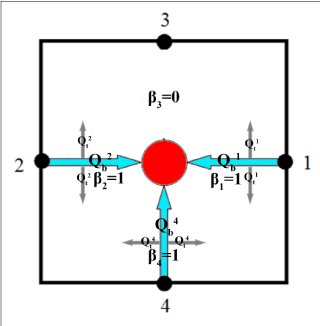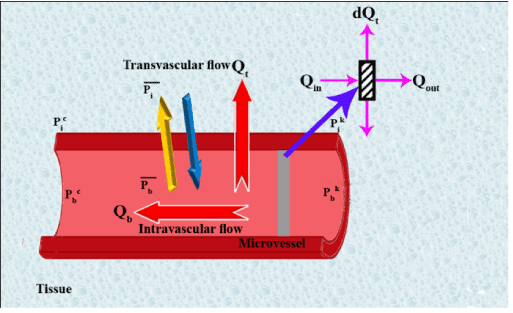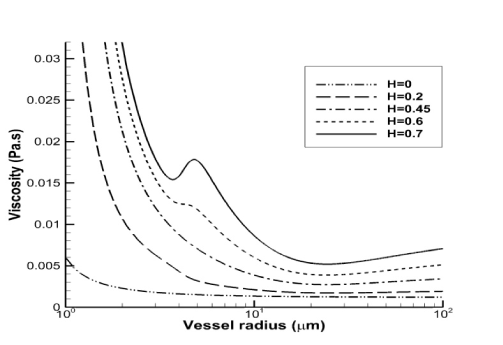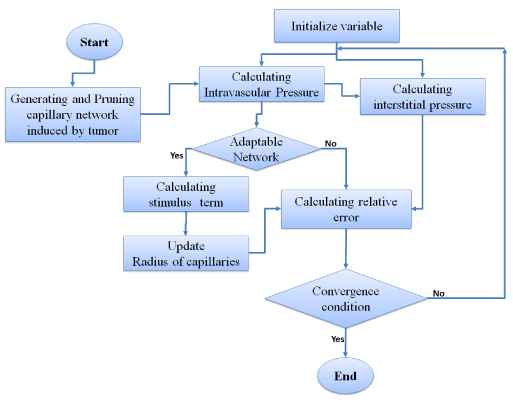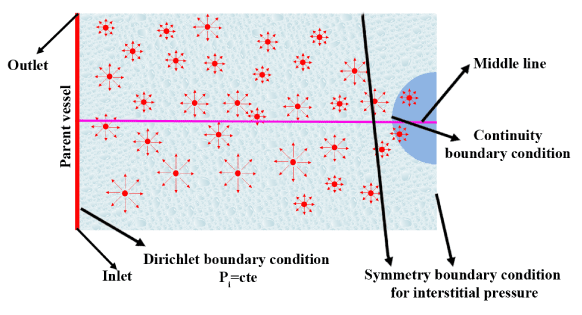Figure 1 Figure 2 Figure 3 Figure 4 Figure 5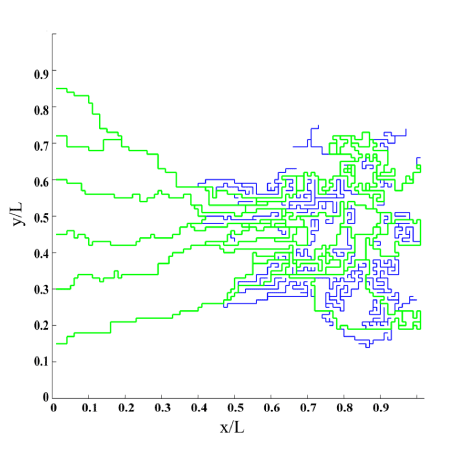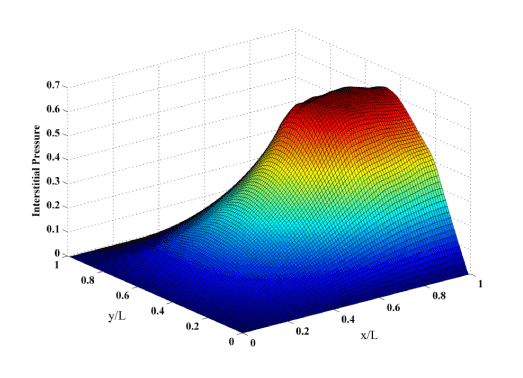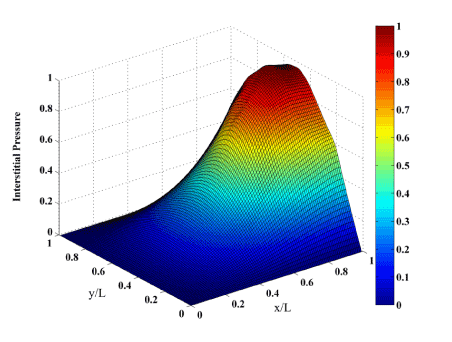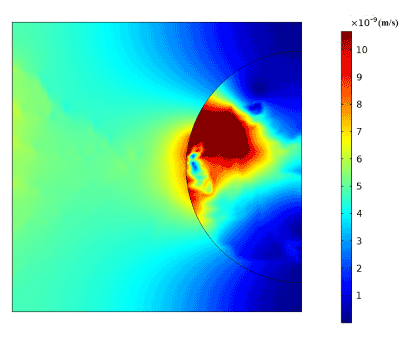Figure 6 Figure 7 Figure 8 Figure 9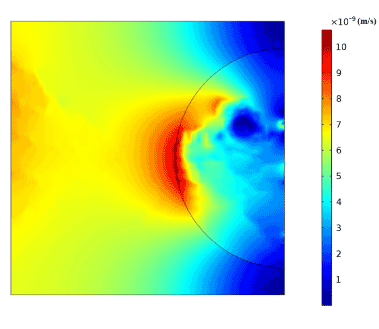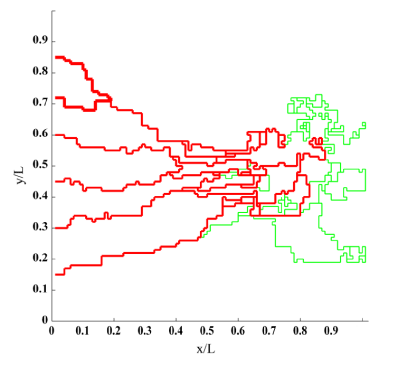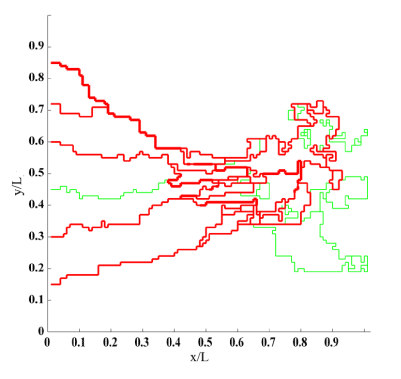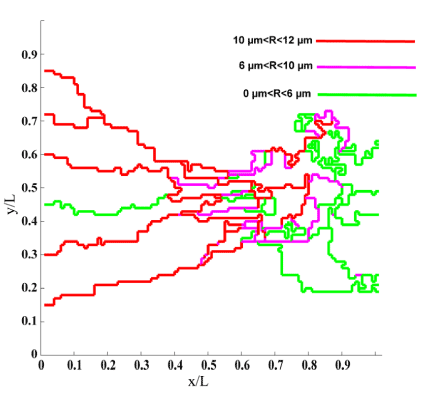Figure 10 Figure 11 Figure 12 Figure 13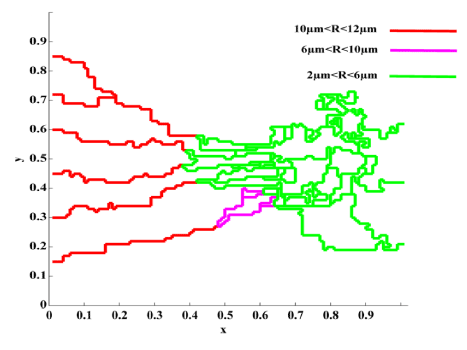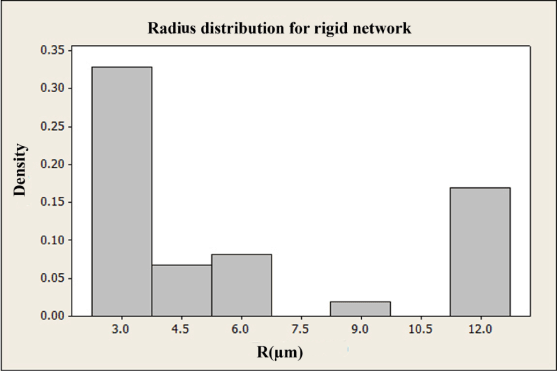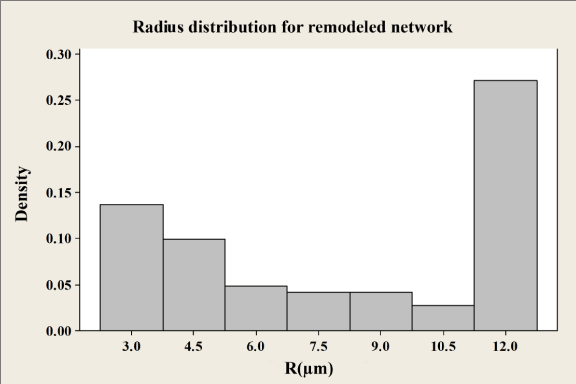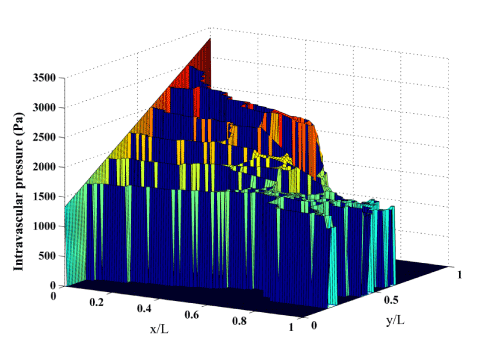Figure 14 Figure 15 Figure 16 Figure 17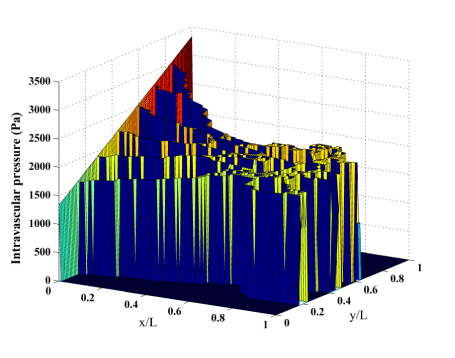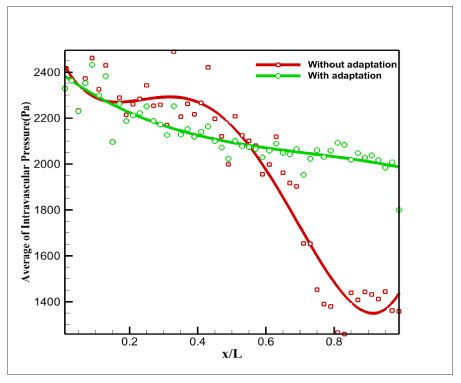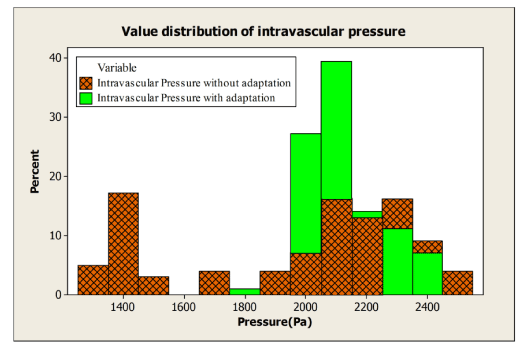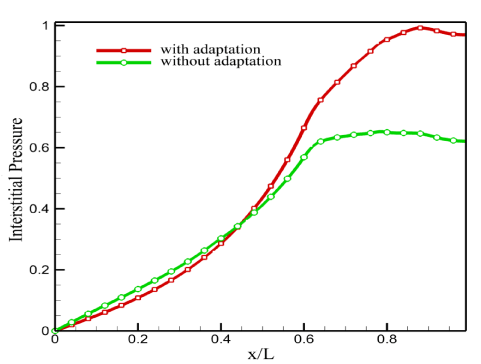Figure 18 Figure 19 Figure 20 Figure 21
Select your language of interest to view the total content in your interested language

### Article Usage

• Total views: 11930
• [From(publication date):
specialissue-2012 - Dec 10, 2019]
• Breakdown by view type
• HTML page views : 8130Can't read the image? click here to refresh